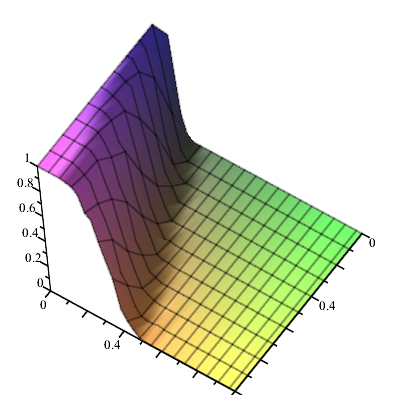:

## All or nothing in graph theory with GraphTheory

Maple

Introduction
The purpose of this post is the investigation of the connection between the connectivity of an undirected graph and the numbers of its vertices and edges with help of the GraphTheory package.
The reader is  referred to http://en.wikipedia.org/wiki/Graph_theory and to ?GraphTheory for info.
Let us create a random graph with 20 vertices and 22 edges and a random graph with 19 vertices and 20 edges :
restart; with(GraphTheory): with(RandomGraphs):randomize():
G := RandomGraph(10, 10):
IsConnected(G);
false

H := RandomGraph(10, 20):
IsConnected(H);
true

Both results are expected. That phenomenon was studied by Paul Erdos and Alfred Renyi (see http://en.wikipedia.org/wiki/Erd%C5%91s%E2%80%93R%C3%A9nyi_model).
I am grateful to Professor Oleh Verbitsky for useful references.
Investigation
We begin from the creation of the sequences of 100 random graphs having k vertices and l edges for different values of k and l. Then we determine the relative frequencies of the connected graphs in those groups.
restart; interface(rtablesize = 30); randomize(); with(GraphTheory): with(LinearAlgebra): with(RandomGraphs): with(Statistics):
S := [seq([seq([seq(RandomGraph(k, l), j = 1 .. 100)], k = 30 .. l, 10)], l = 60 .. 360, 30)];
M := Matrix([seq([seq(evalf((1/100)*nops(select(z -> IsConnected(z), op(j, S[i])))), j = 1 .. nops(S[i]))], i = 1 .. nops(S))]);
Observing the plots
plots:-matrixplot(M, heights = histogram, axes = boxed);
and
plots:-surfdata(convert(M, Array, datatype = float), axes = frame);,

we make the conjecture that this is described by a normal distribution. Let us verify it.
L := Array([seq(seq([NumberOfVertices(S[i, j, 1]), NumberOfEdges(S[i, j, 1]),evalf((1/100)*nops(select(z-> IsConnected(z), op(j, S[i]))))], j = 1 .. nops(S[i])), i = 1 .. nops(S))], datatype = float):
X := Transpose(Matrix(`<,>`(convert(L[() .. (), 1], Vector), convert(L[() .. (), 2], Vector)))):
Y := convert(L[() .. (), 3], Vector):
F := (1+erf(E*b+V*a+c))*(1/2):
I prefer DirectSearch over Statistics because DirectSearch uses a few fit methods versus Statistics.
DirectSearch:-DataFit(F, X, Y, [V, E], initialpoint = [a = 0, b = 0, c = 0], fitmethod = lms);
[HFloat(0.0018873868253531344),                                 [a = HFloat(-.044390558340112664),                              b = HFloat(0.015653645988202017),
c = HFloat(0.6225960328942518)], 649]
A := [seq(RandomGraph(250, 700), n = 1 .. 100)];
evalf((1/100)*nops(z-> IsConnected(z), A)));
0.4300000000
F := unapply(eval(F, [a = -0.443905583401127e-1, b = 0.156536459882020e-1, c = .622596032894252]), V, E);

(V, E) -> 1/2 + 1/2* erf(0.0156536459882020 E - 0.0443905583401127 V
+ 0.622596032894252)
F(250, 700);
0.7524975740
We don't see a good accordance. However, let us continue the investigation.
T := Array(map(c-> F(c, c) end proc, convert(X, listlist)));
R := Array(convert(Y, listlist));
T-R;
If the theory is in accordance with the facts, then the elements of T-R should be normally distributed with the parameters 0 and sigma (see http://en.wikipedia.org/wiki/Regression_analysis ). In view of it
Q := DataSummary(T-R);
[mean = HFloat(0.0011253478765550216),
standarddeviation = HFloat(0.043533760511031055),
skewness = HFloat(0.727348243427046),
kurtosis = HFloat(8.71023319811101),
minimum = HFloat(-0.1624362372000001),
maximum = HFloat(0.2011851919), cumulativeweight = HFloat(209.0)]
ChiSquareSuitableModelTest(T-R, NormalDistribution(0.112534787655502e-2, convert(0.435337605110311e-1, rational)), bins = 8);
hypothesis = false, criticalvalue = HFloat(14.0671405764057),
distribution = ChiSquare(7), pvalue = HFloat(0.0),
statistic = HFloat(145.05904408897578)
A negative result also is a result. The discussion of the topic is welcomed.

RandomGraphs.mw﻿